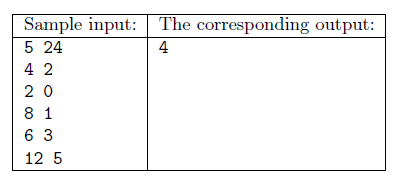Mathematical and Physical Journal
for High Schools
Issued by the MATFUND Foundation
 Already signed up? New to KöMaL?

#Problem I/S. 3. (November 2015)

I/S. 3. One can buy $\displaystyle 1\le N\le 1000$ items in a shop. Your available money is $\displaystyle 1\le P \le 10^9$.

Each item has a price $\displaystyle A_i$, and a home delivery cost $\displaystyle H_i$, so the total cost of item $\displaystyle i$ is $\displaystyle A_i+H_i$ (non-negative integers, $\displaystyle A_i$ is even to simplify this exercise). We also have a coupon to halve the price of a selected item: if we use it with item $\displaystyle i$, then its price would be $\displaystyle A_i/2+H_i$. Determine the maximum number of items that can be bought if we are allowed to use only one coupon. Your program should read the values of $\displaystyle N$ and $\displaystyle P$ from the first line of the standard input, then the integers $\displaystyle A_i$, $\displaystyle H_i$ (separated by a space) from the following $\displaystyle N$ lines. The first and only line of the standard output should contain the maximum number of items that can be purchased.Explanation: it is possible to buy the first 4 items provided that the coupon is used with the third item.

Scoring and bounds. You can get 1 point for a brief and proper documentation clearly describing your solution. Nine further points can be obtained provided that your program solves any valid input within 1 second of running time.

The source code of your program without the .exe or any other auxiliary files generated by the compiler but with a short documentation-also describing which developer environment to use for compiling the source-should be submitted in a compressed file is3.zip.

(10 pont)

Deadline expired on December 10, 2015.

### Statistics:

 25 students sent a solution. 10 points: Alexy Marcell, Bálint Martin, Gergely Patrik, Hornák Bence, Janzer Orsolya Lili, Kiss Gergely, Kovács 246 Benedek, Mernyei Péter, Molnár-Sáska Zoltán, Nagy Ábel, Nagy Nándor, Németh 123 Balázs, Noszály Áron, Radnai Bálint, Zalavári Márton, Zarándy Álmos. 9 points: Szakály Marcell. 8 points: 1 student. 7 points: 2 students. 5 points: 1 student. 3 points: 1 student. 2 points: 1 student. 0 point: 2 students.

Problems in Information Technology of KöMaL, November 2015